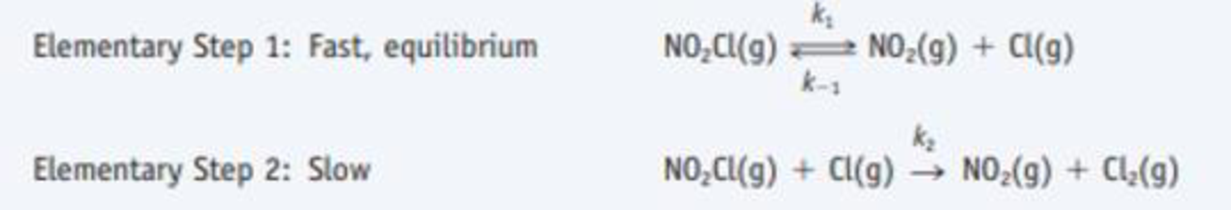# One possible mechanism for the decomposition of nitryl chloride, NO 2 CI, is What is the overall reaction? What rate law would be derived from this mechanism? What effect does increasing the concentration of the product NO 2 have on the reaction rate?### Chemistry & Chemical Reactivity

9th Edition
John C. Kotz + 3 others
Publisher: Cengage Learning
ISBN: 9781133949640

#### Solutions

Chapter
Section### Chemistry & Chemical Reactivity

9th Edition
John C. Kotz + 3 others
Publisher: Cengage Learning
ISBN: 9781133949640
Chapter 14.6, Problem 3CYU
Textbook Problem
107 views

## One possible mechanism for the decomposition of nitryl chloride, NO2CI, isWhat is the overall reaction? What rate law would be derived from this mechanism? What effect does increasing the concentration of the product NO2 have on the reaction rate?

Interpretation Introduction

Interpretation: The rate law, overall reaction, effect of increasing concentration of the product on reaction rate has to be given.

Concept Introduction:

The rate of reaction is the quantity of formation of product or the quantity of reactant used per unit time.  The rate of reaction doesn’t depend on the sum of amount of reaction mixture used.

The raise in molar concentration of product of a reaction per unit time or decrease in molarity of reactant per unit time is called rate of reaction and is expressed in units of mol/(L.s) .

The variation in concentration of reaction or product over a certain interval of time is called average reaction rate.

The equation that relates the reaction rate to the reactants concentrations that is raised to various powers is called as rate law.

Rate law can be determined by the slow step or otherwise called as rate-determining step.

### Explanation of Solution

The overall reaction rate law and the effect is given as,

Overall reaction:

Step1:fast,equilibriumNO2Cl(g)  k-1k1NO2(g) + Cl(g)Step2:slowNO2Cl(g) + Cl(g) k2NO2(g) + Cl2(g)_overallreaction:2NO2Cl(g)     ®2NO2(g)   + Cl2(g)_

The overall rate of the given chemical reaction is k[NO]2[O2]

Overall rate of reaction:

Step1:fast,equilibriumNO2Cl(g)  k-1k1NO2(g) + Cl(g)Step2:slow

### Still sussing out bartleby?

Check out a sample textbook solution.

See a sample solution

#### The Solution to Your Study Problems

Bartleby provides explanations to thousands of textbook problems written by our experts, many with advanced degrees!

Get Started

Find more solutions based on key concepts
Digestible carbohydrates are absorbed as through the small intestinal wall and are delivered to the liver, whic...

Nutrition: Concepts and Controversies - Standalone book (MindTap Course List)

What wind system flows along the northern margin?

Fundamentals of Physical Geography

Sieve tubes are part of ______. a. cortex b. mesophyll c. phloem d. xylem

Biology: The Unity and Diversity of Life (MindTap Course List)

The kinetic energy of an object is increased by a factor of 4. By what factor is the magnitude of its momentum ...

Physics for Scientists and Engineers, Technology Update (No access codes included)

How do you recognize the formula of an acid?

Introductory Chemistry: An Active Learning Approach